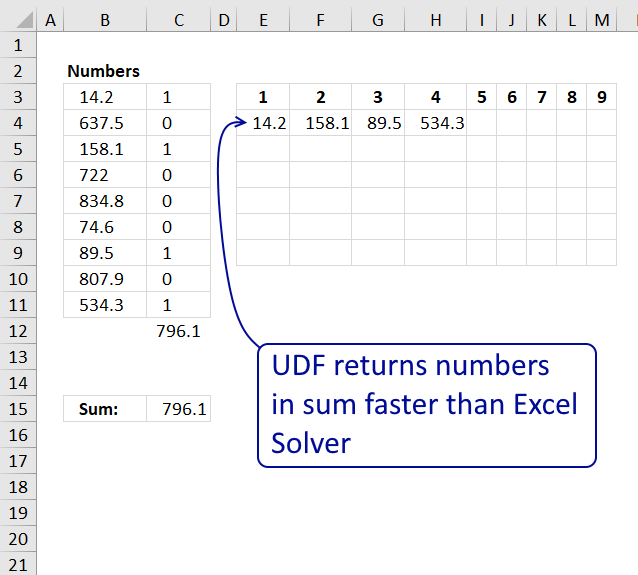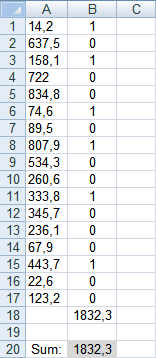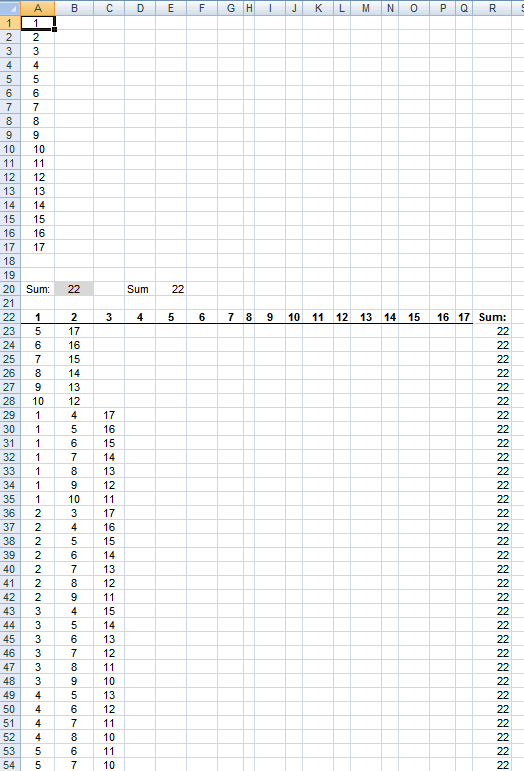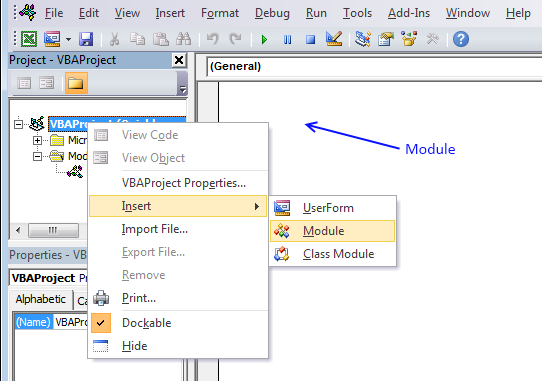Author: Oscar Cronquist Article last updated on April 30, 2019This article describes how to find a sum from a range of numbers using a user defined function.

Let´s see how quickly excel solver finds the numbers using the instructions from this post: Identify numbers in sum using solver in excel.The sum is 1832,3 and it takes around 15 seconds to find the numbers.

With my beginner vba skills I created an user defined function to find numbers in a sum. See picture below.The udf finds two sets of numbers in a second.

UDF in A23:Q25:

=Find_num(A1:A17, B20)

To enter an array formula, type the formula in a cell then press and hold CTRL + SHIFT simultaneously, now press Enter once. Release all keys.

The formula bar now shows the formula with a beginning and ending curly bracket telling you that you entered the formula successfully. Don't enter the curly brackets yourself.

Formula in R23 sums the numbers. Here is another example:User defined function:

```Function Find_num(rng As Range, cell As Range)

Dim com() As Single, c As Single, i As Single
Dim s As Single, d As Single, u As Single, v As Single
Dim arr() As String, r As Single, p As Single, t As Single
Dim sum_cells As Single, j As Single, k As Single, l As Single

sum_cells = Application.WorksheetFunction.Sum(rng)
c = rng.Rows.Count
ReDim arr(1 To 1000, 1 To c)
r = 1

For i = 0 To Int(c / 2)
If i <> Int(c / 2) - 1 Then
t = WorksheetFunction.Combin(c, i + 1)
Else
t = WorksheetFunction.Combin(c, i + 1) / 2
End If
ReDim com(i)
For j = 0 To i
com(j) = j + 1
Next
k = i
For s = 1 To t
If com(k) > c And i > 0 Then
p = 0

Do Until com(k) <= c - p
com(k - 1) = com(k - 1) + 1
k = k - 1
p = p + 1
Loop

Do Until k >= i
k = k + 1
com(k) = com(k - 1) + 1
Loop

End If
d = 0

For j = 0 To i
d = d + rng(com(j))
Next j

If d = cell Then

For j = 1 To i + 1
arr(r, j) = rng(com(j - 1))
Next j

r = r + 1
End If

If sum_cells - d = cell Then

For j = 1 To c
v = 0

For u = 0 To i
If rng(com(u)) = rng(j) Then v = 1
Next u

If v = 0 Then
arr(r, j) = rng(j)
End If

Next j
r = r + 1

End If
com(k) = com(k) + 1
Next s
Next i

Find_num = arr()

End Function

```1. Press Alt-F11 to open visual basic editor
2. Click Module on the Insert menu
3. Copy code above
4. Paste code to code module